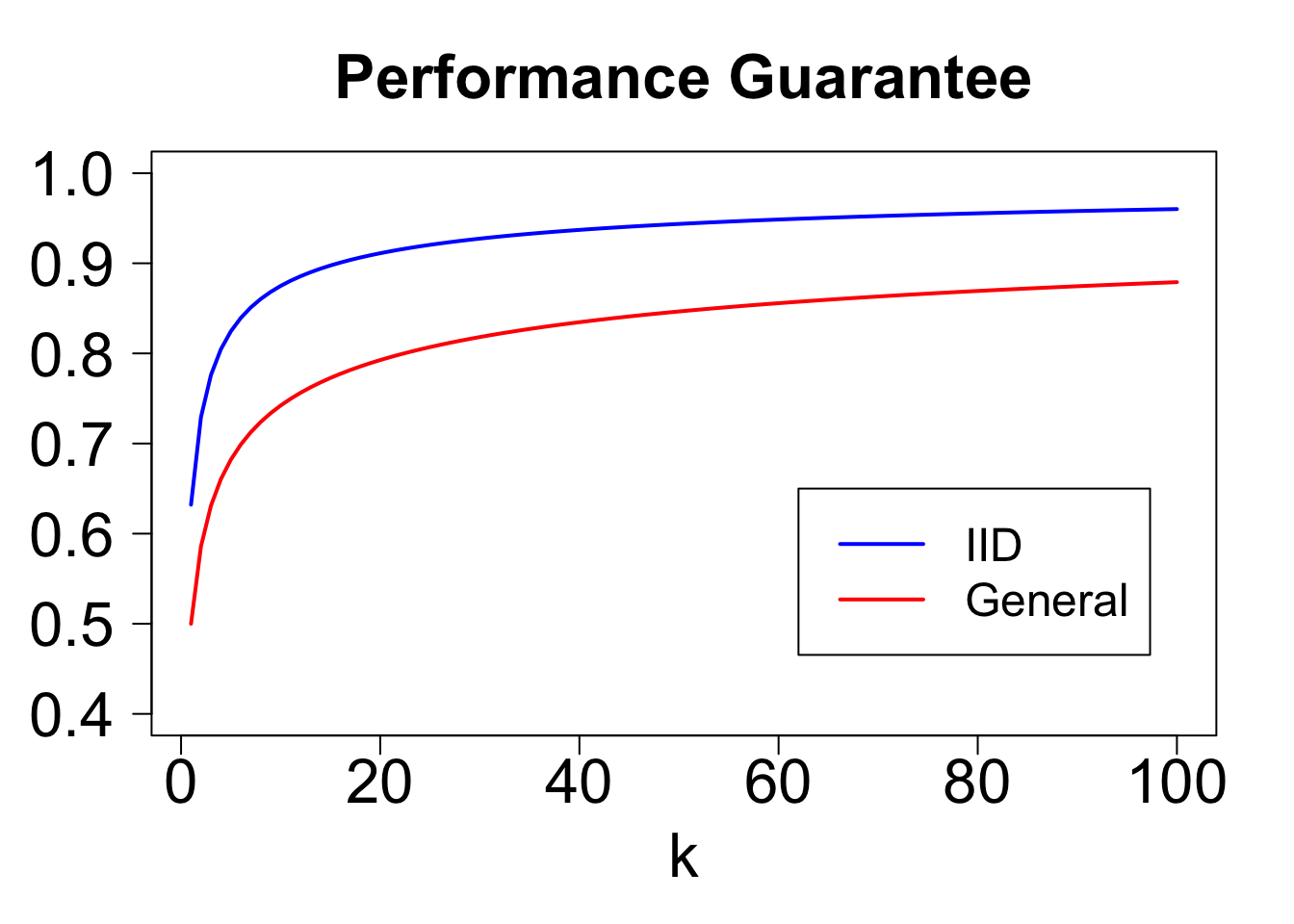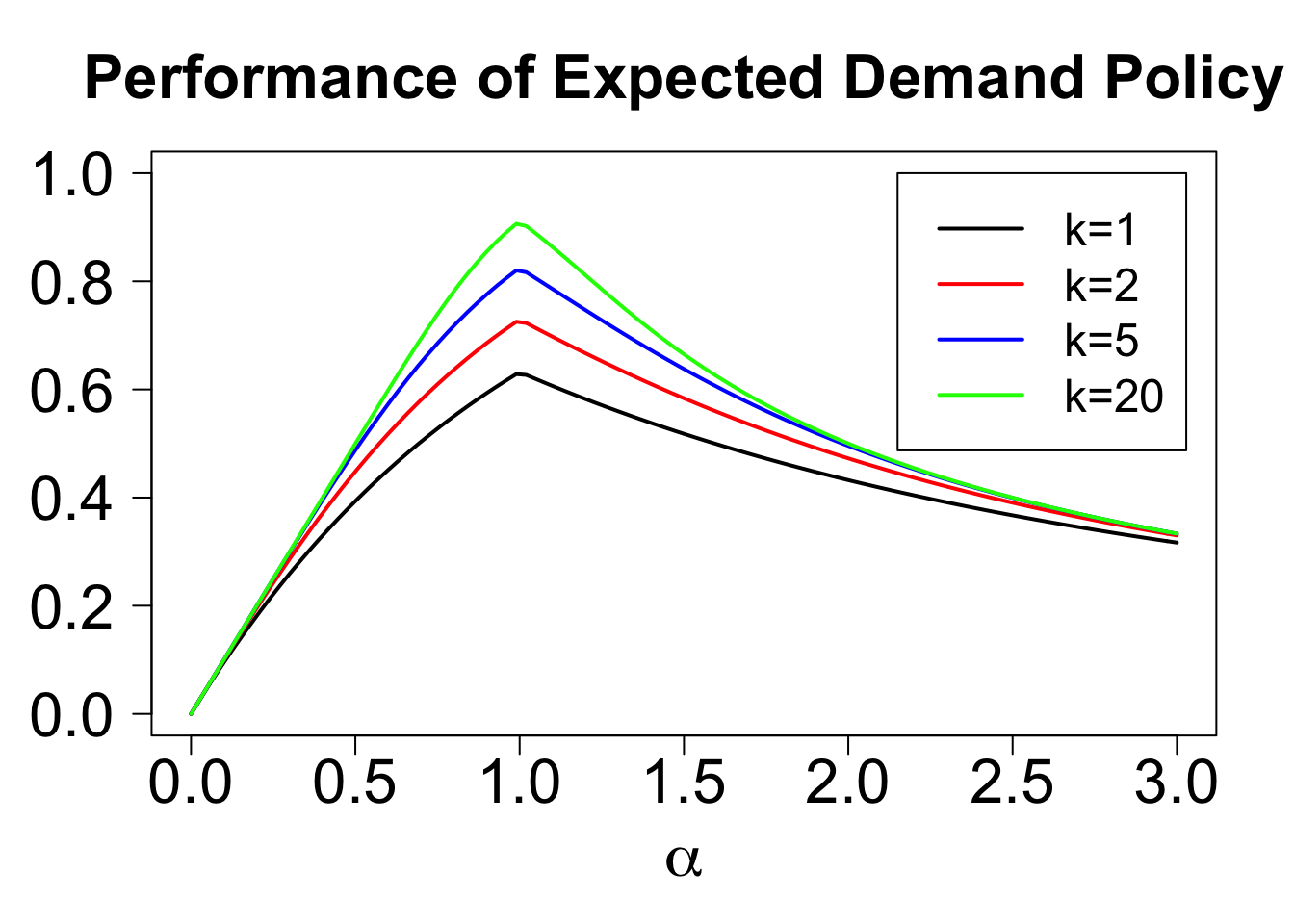# IID Prophet Inequalities

## 2020/10/02

Last week I had the pleasure of seeing Nikhil Devanur present Static pricing for multi-unit prophet inequalities, which is joint with Thodoris Lykouris and Shuchi Chawla. I greatly enjoyed the paper, and discussed it in a blog post last week. Today, I will go beyond what is in their paper to tackle a question asked by my colleague Will Ma.

# 1 Setting the Stage

The original paper considers a setting where we are presented with a sequence of values. Each value $$V_i$$ is drawn from a known distribution $$F_i$$. We are allowed to "keep" at most $$k$$ values, and wish to maximize the sum of kept values. We will use a simple static threshold algorithm, which keeps the first $$k$$ values above some fixed threshold $$t$$. We wish to benchmark the performance of this algorithm against that of an omniscient benchmark that always keeps the $$k$$ highest values. The original paper provides an algorithm for choosing $$t$$, and a tight analysis of its performance. The following code computes its competitive ratio, as a function of $$k$$ (see my preceding post for more explanation):

delta_pois = function(lambda,k){return(ppois(k-1,lambda))}
mu_pois = function(lambda,k){
prob = dpois(0:(k-1),lambda)
prob = c(prob,1-sum(prob))
return(sum(prob*c(0:k))/k)
}
guarantee_at_lambda = function(k){
return(Vectorize(function(lambda){
return(min(delta_pois(lambda,k),mu_pois(lambda,k)))
}))
}
guarantee_pois = Vectorize(function(k){
return(optimize(guarantee_at_lambda(k),interval=c(0,k),maximum=TRUE)\$objective)
})
round(guarantee_pois(c(1:10)),3)
##   0.500 0.586 0.631 0.660 0.682 0.699 0.713 0.724 0.734 0.742

This post will consider a simpler setting where the $$F_i$$ are iid. This should be an easier problem, so we might hope to get a better guarantee. However, the guarantee of their algorithm does not improve! Their algorithm sets a threshold such that the expected number of items kept divided by $$k$$ is equal to the guarantee computed above. Therefore, if all values are "nearly deterministic" (i.e. $$U[1-\epsilon,1+\epsiloin]$$), its performance will be approximately equal to their guarantee.

In this post, I show the following:

1. We can get a better guarantee by using a threshold such that the expected "demand" (number of values exceeding the threshold) is equal to $$k$$.
2. This algorithm provides the best guarantee among the natural class of "expected demand" policies.

# 2 The LP Approximation Policy

Their policy's performance is hurt by the fact that it sets too high of a threshold (keeps too few items). To get a better guarantee, we must set a lower threshold, but how much lower?

In the previous post we defined the "demand" at $$t$$ to be the number of values exceeding $$t$$: $D_t(V) = \sum_{i = 1}^n {\bf 1}(V_i > t).$ We'll start by considering a natural policy: use the threshold $$t^{LP}$$ such that the expected demand is equal to $$k$$. We can analyze its performance as follows.

For any threshold $$t$$, we have $$D_t \sim Binom(n,1-F(t)).$$ We keep $$\min(D_t(V),k)$$ values, each with expected value $$\mathbb{E}_{V \sim F}[V \vert V > t]$$. Meanwhile, the LP relaxation discussed in my previous post gives an upper bound of $$k \cdot \mathbb{E}_{V \sim F}[V \vert V > t^{LP}]$$. Combining these, we get the following performance guarantee: $\frac{ST_k^{t^{LP}}(F)}{LP_k(F)} = \frac{\mathbb{E}[\min(D_{t^{LP}}(V),k)] \cdot\mathbb{E}_{V \sim F}[V \vert V > t^{LP}]}{k\cdot \mathbb{E}_{V \sim F}[V \vert V > t^{LP}]}\hspace{.3 in} = \frac{\mathbb{E}[\min(Binom(n,k/n),k)]}{k}.$

For any $$k$$, this expression falls as $$n$$ grows. In the limit as $$n \rightarrow \infty$$, the Binomial becomes a Poisson, yielding the following bounds.

mu_binom = function(n,k,p){
prob = dbinom(0:(k-1),n,p)
prob = c(prob,1-sum(prob))
return(sum(prob*c(0:k))/k)
}
iid_guarantee = Vectorize(function(n,k){return(mu_binom(n,k,k/n))}) #For finite k, n
iid_guarantee_pois = Vectorize(function(k){return(mu_pois(k,k))}) #Limit as n -> \infty
round(iid_guarantee_pois(c(1:10)),3)
##   0.632 0.729 0.776 0.805 0.825 0.839 0.851 0.860 0.868 0.875

Let's compare these to the bounds obtained for the general case. For $$k = 1$$, the bound improves from $$1/2$$ to $$1 - 1/e$$. Even for very large $$k$$, there remains a sizeable gap. For example, with $$k = 100$$, the general bound is $$0.88$$, and the iid bound is $$0.96$$.

kVals = c(1:100)
colors = c('blue','red')
plot(NULL,xlim=c(min(kVals),max(kVals)),ylim=c(0.4,1),las=1,xlab='k',ylab='',main='Performance Guarantee',cex.axis=2,cex.lab=2,cex.main=2)
lines(kVals,iid_guarantee_pois(kVals),col=colors,lwd=2)
lines(kVals,guarantee_pois(kVals),col=colors,lwd=2)
legend(.62*max(kVals),.65,col=colors,lty=c('solid','solid'),lwd=2,legend = c('IID','General'),cex=1.5)# 3 Expected Demand Policies

The preceding analysis establishes a performance guarantee for the threshold $$t^{LP}$$. But is this threshold optimal? For most $$F$$, it is not. However, in we will show that this selection provides the best worst-case guarantee among the following subset of static threshold policies.

Expected Demand Policy. Given $$\alpha > 0$$, choose a threshold $$t$$ such that expected demand $$n(1 - F(t))$$ is equal to $$\alpha \cdot k$$.

We denote the performance of this policy on distribution $$F$$ by $$ED_k^{\alpha}({\bf F})$$.

One way to think of expected demand policies is that they specify the threshold as a fixed quantile of the distribution $$F$$. If the quantile can depend on $$n, k,$$ and $$F$$, then the set of expected demand policies coincides with the set of static threshold policies. However, we will ask the question, can we choose an $$\alpha$$ that works well simultaneously for all $$n,k,F$$? To start, we fix $$n$$ and $$k$$, and solve the following problem: $\sup \limits_\alpha \inf \limits_{F} \frac{ED_k^\alpha(F)}{LP_k(F)}.$

Our preceding analysis was for $$\alpha = 1$$. It turns out that no other choice of $$\alpha$$ offers a better guarantee.

The intuition is as follows. If $$\alpha < 1$$, the guarantee will be poor if values are nearly deterministic (we will keep too few values). If $$\alpha > 1$$, the guarantee will be poor if the distribution puts a tiny amount of weight on an immense value (we will too often reach our quota and miss out on a very high value).

We can formalize this as follows. Let $$B_{n,p}$$ denote a binomial with parameters $$n$$ and $$p$$. For any $$\alpha < 1$$, let $$F$$ be uniform on $$[1-\epsilon,1+\epsilon]$$. As $$\epsilon \rightarrow 0$$, our competitive ratio converges to the expected number of values we keep, divided by $$k$$: $\frac{ED_k^\alpha(F)}{OPT_k(F)} \rightarrow \frac{\mathbb{E}[\min(B_{n,\alpha\cdot k/n},k)]}{k}$

For any $$\alpha > 1$$, let $$F$$ be such that $$v_i$$ is $$1/\epsilon^2$$ with probability $$\epsilon$$, and otherwise is uniform on $$[0,1]$$. If $$\epsilon$$ is small, all that really matters is how often we keep large values. The optimal policy will save spots for a large value, whereas the $$ED_k^\alpha$$ policy will sometimes run out of room. In particular, as $$\epsilon \rightarrow 0$$, the competitive ratio will converge to $\frac{ED_k^\alpha(F)}{OPT_k(F)}= \frac{\mathbb{E}[\min(B_{n,\alpha\cdot k/n},k)]}{\alpha k}.$

With a little work, we can show that for any $$\alpha$$, one of these families of distributions is the worst case, so the competitive ratio for the expected demand policy with parameter $$\alpha$$ is $\frac{\mathbb{E}[\min(B_{n,\alpha \cdot k/n},k)]}{\max(\alpha,1)\cdot k}.$

As $$n$$ grows, this bound falls, and the binomial converges to a Poisson. We can therefore plot the worst-case performance of an expected demand policy as a function of $$\alpha$$ and $$k$$. For all $$k$$, the optimal choice of $$\alpha$$ is one, and the guarantee offered by this policy improves with $$k$$.

iid_guarantee_alpha = Vectorize(function(alpha,k){return(mu_pois(alpha*k,k)/max(alpha,1))})
alphaMax = 3
plot(NULL,las=1,xlim=c(0,alphaMax),ylim=c(0,1),xlab=expression(alpha),ylab='',main='Performance of Expected Demand Policy',cex.lab=2,cex.axis=2,cex.main=2)
k = c(1,2,5,20)
colors = c('black','red','blue','green')
for(i in 1:length(k)){
legend(2.15,1,col=colors,lty=rep('solid',length(k)),lwd=2,legend=c('k=1','k=2','k=5','k=20'),cex=1.5)This post has showed when values are iid, a very simple policy achieves a significant improvement over the guarantees that are known for the non-iid case. While this policy offers the best guarantee of any "expected demand" policy, I don't know whether the resulting bounds are tight for the class of static threshold policies. It would be interesting to see if there is a distribution $$F$$ for which no choice of threshold offers a better guarantee.